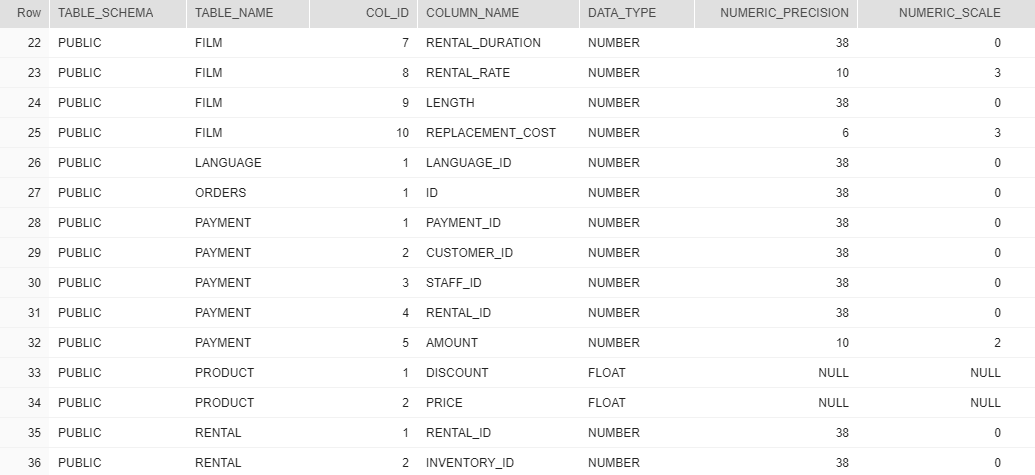## Find all numeric columns in Snowflake

Article for:

In snowflake almost all numeric types are aliases to NUMBER type with diffent precision and scale. Types in Snowflake:

• DECIMAL, NUMERIC are aliases to NUMBER

• INT, INTEGER, BIGINT, SMALLINT, TINYINT, BYTEINT are aliases to NUMBER(38, 0)

• FLOAT, FLOAT4, FLOAT8 - Snowflake uses double-precision (64 bit) IEEE 754 floating point numbers.

• DOUBLE, DOUBLE PRECISION, REAL - synonymous to FLOAT

## Query

``````select col.table_schema,
col.table_name,
col.ordinal_position as col_id,
col.column_name,
col.data_type,
col.numeric_precision,
col.numeric_scale
from information_schema.columns col
join information_schema.tables tab on tab.table_schema = col.table_schema
and tab.table_name = col.table_name
and tab.table_type = 'BASE TABLE'
where col.data_type in ('NUMBER', 'FLOAT')
order by col.table_schema,
col.table_name,
col.ordinal_position;
``````

## Columns

• table_schema - name of the schema
• table_name - name of the table
• column_id - column position in table
• column_name - name of the column
• data_type - type of data
• numeric_precision - precision of the column
• numeric_scale - scale of column

## Rows

• One row represents one column with a numeric data type
• Scope of rows: all columns containing numeric data types in the database (schema)
• Ordered by table schema name and table name

## Sample results